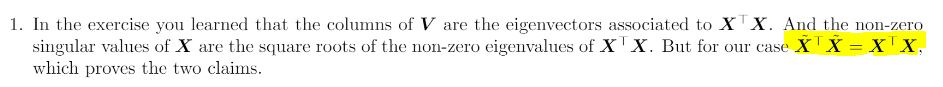### Problem 24 first question

Hi I don't understand why the last matrix inequality in the solution holds? Is this a property of a gram matrix?

Thanks!I don't understand in general what is the logic we should use in this exercise. Could someone explain more clearly the solution? It is very schematic and not very clear to me. Thanks!

Start by noticing that since X is a DxN matrix, both $$X^T X$$ and $$\tilde{X}^T \tilde{X}$$ are NxN matrix. Prove the equality element-wise:
$$(X^T X)_{ij} = \Sigma_{k=1}^{D} (X^T)_{ik}X_{kj} = \Sigma_{k=1}^{D-1} x_{ki}x_{kj} + x_{Di} x_{Dj}$$
and $$(\tilde{X}^T \tilde{X})_{ij} = \Sigma_{k=1}^{D-1} \tilde{x}_{ki}x_{kj} + \tilde{x}_{Di} \tilde{x}_{Dj} + \tilde{x}_{(D+1)i} \tilde{x}_{(D+1)j}$$
Then remember $$X$$ is normalized everywhere with subtracted mean and variance except that its last row has variance 2 while $$\tilde{X}$$ is normalized in every row, and that its last row is equal to its penultimate row. That means that we can rewrite:
$$(\tilde{X}^T \tilde{X})_{ij} = \Sigma_{k=1}^{D-1} x_{ki}x_{kj} + \frac{x_{Di}}{\sqrt{2}} \frac{x_{Dj}}{\sqrt{2}} + \frac{x_{Di}}{\sqrt{2}} \frac{x_{Dj}}{\sqrt{2}}$$ which reduces to $$(X^T X)_{ij}$$.

Page 1 of 1